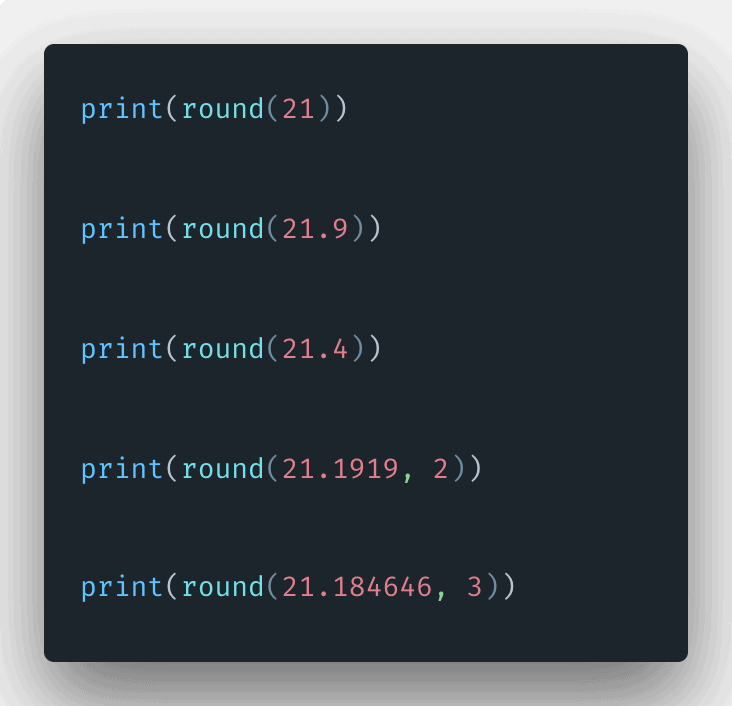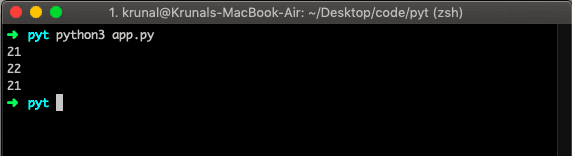# Python round: How to Use round() Function in Python

0
8Python round() is an inbuilt function that returns the floating-point number rounded off to the given digits after the decimal point. The round() method rounds off to a given number of digits and returns a floating-point number, if no number of digits is provided for round off, it rounds off a number to the nearest integer.

## Python Round Example

Python round() function returns the floating-point number that is the rounded version of a specified number, with a specified number of decimals.

The syntax of the Python round() function is the following.

`round(number, digits)`

The round() method takes two parameters:

• number – the number that is to be rounded.
• ndigits (Optional) – the number up to which the given number is to be rounded.

See the below example.

```# app.py

# for integers
print(round(21))

# for floating point
print(round(21.9))

# even choice
print(round(21.4))```

See the output.## Round a number to given ndigits places

See the example below.

```# app.py

print(round(21.1919, 2))

print(round(21.184646, 3))
```

In the above example, we have passed the second argument which is up to how many digits, you need to round up. In the first example, it is 2, and in the second example, it is 3.

The best use case of functions is handling the mismatch between fractions and decimals.

So, Python round()  function is a built-in function in Python. It returns x rounded to n digits from the decimal point. Finally, Python Round Example is over. Thanks.

This site uses Akismet to reduce spam. Learn how your comment data is processed.